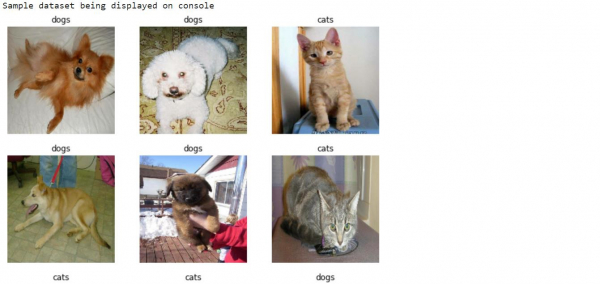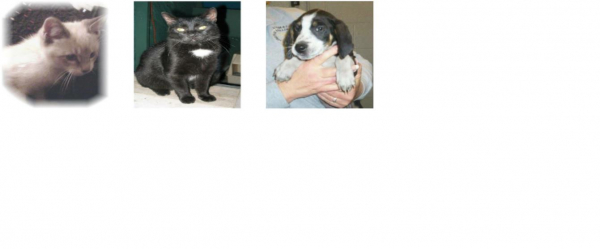# How can Tensorflow be used to display sample data from the cats and dogs input dataset?

TensorflowServer Side ProgrammingProgramming

Tensorflow can be used to display sample data from the cats and dogs input dataset with the help of ‘matplotlib’ library. The ‘imshow’ method can be used to display the images on the console.

We will understand how to classify images of cats and dogs with the help of transfer learning from a pre-trained network.

The intuition behind transfer learning for image classification is, if a model is trained on a large and general dataset, this model can be used to effectively serve as a generic model for the visual world. It would have learned the feature maps, which means the user won’t have to start from scratch by training a large model on a large dataset.

We are using the Google Colaboratory to run the below code. Google Colab or Colaboratory helps run Python code over the browser and requires zero configuration and free access to GPUs (Graphical Processing Units). Colaboratory has been built on top of Jupyter Notebook.

## Example

class_names = train_dataset.class_names
print("Sample dataset being displayed on console")
plt.figure(figsize=(10, 10))
for images, labels in train_dataset.take(1):
for i in range(9):
ax = plt.subplot(3, 3, i + 1)
plt.imshow(images[i].numpy().astype("uint8"))
plt.title(class_names[labels[i]])
plt.axis("off")

## Output## Explanation

• The images are iterated over and a sample of the images are displayed on the console.